### Home > CAAC > Chapter 10 > Lesson 10.1.1 > Problem10-11

10-11.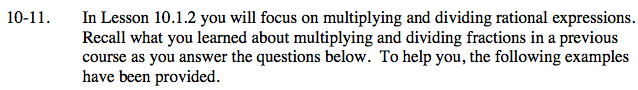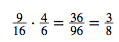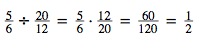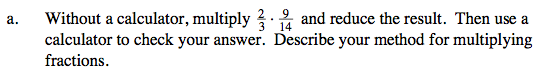After multiplying all numbers in the numerator and the denominator, look for factors that simplify to one.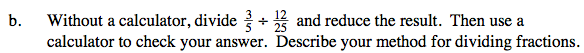$\frac{3}{5}\left(\frac{25}{12}\right)$

Rewrite the expression as a product.

$\frac{75}{60}$

Multiply the numerators and the denominators to find their product.

$\frac{5}{4}$